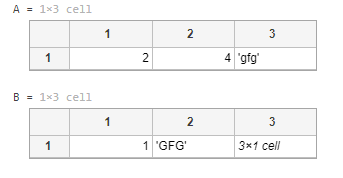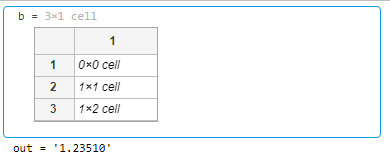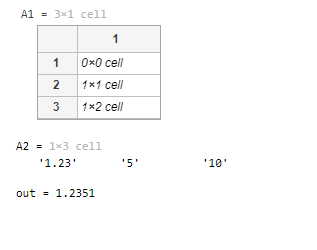Related Articles

# How to extract numbers from cell array in MATLAB?

• Last Updated : 23 Jul, 2021

In this article, we are going to discuss the extraction of numbers from the cell array with the help of regexp(), str2double(), cat(), and isletter() functions.

## What is aCell Array?

A cell array is nothing but a data type having indexed data containers called cells, where each cell contains any type of data. It mainly contains either a list of texts, combinations of text and numbers, or numeric arrays of different sizes.

Example:

## Matlab

 `% MATLAB code for put data in the cell array``A = {2, 4, ``'gfg'``}``B = {1, ``'GFG'``, {5; 10; 15}}`

Output:## Using regexp( )

The regexp() function is used for matching the regular expression. It is case-sensitive.

Syntax:

startIndex = regexp(str, expression)

[startIndex, endIndex] = regexp(str, expression)

out = regexp(str, expression, outkey)

• startIndex = regexp(str, expression) is used to return the starting index of each substring of str that matches the character patterns specified by the regular expression. If there are no matches, startIndex is an empty array.
• [startIndex,endIndex] = regexp(str,expression) is used to return the starting and ending indices of all matches.
• regexp(str, expression, outkey) is used to return the output specified by the outkey. For example, if outkey is ‘match’, then this function returns the substrings that match the expression rather than their starting indices.

Example:

## Matlab

 `% MATLAB code for regexp with strjoin()``% Initializing a cell array``A = {``'gfg'``; ``'gfg1.23GFG'``; ``'5gfg10'``};``b=regexp(A,``'\d+(\.)?(\d+)?'``,``'match'``)``out=strjoin([b{:}],``''``)`

Output:## Using str2double( )

The str2double() function is used for the conversion of strings to double-precision values.

Syntax:

str2double(string)

Here, str2double(string) is used to convert the text in the specified string to double-precision values.

Example:

## Matlab

 `% MATLAB code for regexp() demonstration``% Initializing a cell array``A = {``'gfg'``; ``'gfg1.23GFG'``; ``'5gfg10'``};`` ` `% Calling the regexp() function over the``% above cell array to extract number part``B = regexp(A,``'\d+(\.)?(\d+)?'``,``'match'``);`` ` `% Calling the str2double() function to ``% convert the text to double-precision values``out = str2double([B{:}])`

Output:

```out =
1.2300    5.0000   10.0000```

## Using cat()

The cat() function is used to concatenate the specified arrays.

Syntax:

cat(dim, A, B)

cat(dim, A1, A2 ,…, An)

• cat(dim, A, B) is used to concatenate “B” to the end of “A” along with the dimension “dim” when A and B have compatible sizes, i.e. the lengths of the dimensions match except for the operating dimension dim.
• cat(dim, A1, A2, …, An) is used to concatenate “A1, A2, …, An” along with the dimension dim.

## Matlab

 `% MATLAB code for extract numbers from``% cell using regexp with strcat()``% Initializing a cell array``A = {``'gfg'``; ``'gfg1.23GFG'``; ``'5gfg10'``};``A1 = regexp(A,``'[\d*\.]*\d*'``,``'match'``)``A2 = [A1{:}]``out = str2double(strcat(A2{:}))`

Output:## Using isletter()

The isletter() function is used to find the array of elements that are letters of the alphabet.

Syntax:

isletter(‘string’)

Here, isletter(‘string’) is used to return an array the same size as the specified “string” that contains logical true i.e. 1 when the elements of “string” are letters of the alphabet, and logical false i.e. 0 when they are not.

Example

## Matlab

 `% MATLAB code for isletter() demonstration``% Initializing a cell array``A = {``'gfg'``; ``'gfg1.23GFG'``; ``'5gfg'``};`` ` `% Calling the cat() function to``% concatenate the data of the above``% cell array into a single string``% one after another``S = cat(2, A{:});`` ` `% Calling the isletter() function``% to filter the numeric value ``S(isletter(S)) = []`

Output:

`S = 1.235`

My Personal Notes arrow_drop_up